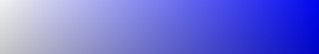﻿ Python生成字符视频的实现示例_python_脚本之家
python# Python生成字符视频的实现示例

## 一、前言## 二、OpenCV的操作图像

`pip install opencv-python`

### 2.1、读取和显示

```import cv2
# 读取图片
# 显示图片
cv2.imshow("img", img)
cv2.waitKey()
cv2.destroyAllWindows()
```

### 2.2、灰度转换

```import cv2
# 灰度转换
gray = cv2.cvtColor(img, cv2.COLOR_BGR2GRAY)
```

```import cv2
# 以灰度形式读入
```

### 2.4、获取图片尺寸并修改尺寸

```import cv2
# 获取图片的高宽
h, w = img.shape
# 缩放图片
res = cv2.resize(img, (w//2, h//2))
```

### 2.5、绘制文字

```import cv2
# 绘制文字
cv2.putText(
# 背绘制的图片
img,
# 要绘制的文字
'Hello',
# 文字左下角的坐标
(100, 500),
# 字体
cv2.FONT_HERSHEY_SIMPLEX,
# 字体大小缩放
20,
# 文字颜色
(0, 0, 0),
# 文字粗细
10
)```

### 2.6、读取视频

```import cv2
# 读取视频
cap = cv2.VideoCapture('1.mp4')
# 获取视频的帧率
fps = cap.get(cv2.CAP_PROP_FPS)
# 循环读取图片的每一帧
while True:
# 读取下一帧
if not ret:
break
else:
pass
cap.release()
```

### 2.7、写入视频

```import cv2
fourcc = cv2.VideoWriter_fourcc(*'mp4v')
writer = cv2.VideoWriter('11.mp4', fourcc, fps, (w, h))
# 写入视频
writer.write(frame)
***
write.release()
```

## 三、像素映射成字符mqpka89045321@#\$%^&*()_=||||}```def pixel2char(pixel):
char_list = "@#\$%&erytuioplkszxcv=+---.     "
index = int(pixel / 256 * len(char_list))
return char_list[index]
```

## 四、生成字符图片

```def get_char_img(img, scale=4, font_size=5):
# 调整图片大小
h, w = img.shape
re_im = cv2.resize(img, (w//scale, h//scale))
# 创建一张图片用来填充字符
char_img = np.ones((h//scale*font_size, w//scale*font_size), dtype=np.uint8)*255
font = cv2.FONT_HERSHEY_SIMPLEX
# 遍历图片像素
for y in range(0, re_im.shape):
for x in range(0, re_im.shape):
char_pixel = pixel2char(re_im[y][x])
cv2.putText(char_img, char_pixel, (x*font_size, y*font_size), font, 0.5, (0, 0, 0))
return char_img
```

```import cv2
import numpy as np

def pixel2char(pixel):
char_list = "@#\$%&erytuioplkszxcv=+---.     "
index = int(pixel / 256 * len(char_list))
return char_list[index]

def get_char_img(img, scale=4, font_size=5):
# 调整图片大小
h, w = img.shape
re_im = cv2.resize(img, (w//scale, h//scale))
# 创建一张图片用来填充字符
char_img = np.ones((h//scale*font_size, w//scale*font_size), dtype=np.uint8)*255
font = cv2.FONT_HERSHEY_SIMPLEX
# 遍历图片像素
for y in range(0, re_im.shape):
for x in range(0, re_im.shape):
char_pixel = pixel2char(re_im[y][x])
cv2.putText(char_img, char_pixel, (x*font_size, y*font_size), font, 0.5, (0, 0, 0))
return char_img

if __name__ == '__main__':
res = get_char_img(img)
cv2.imwrite('d.jpg', res)

```## 五、生成字符视频

```def generate(input_video, output_video):
# 1、读取视频
cap = cv2.VideoCapture(input_video)

# 2、获取视频帧率
fps = cap.get(cv2.CAP_PROP_FPS)

# 读取第一帧，获取转换成字符后的图片的尺寸
char_img = get_char_img(cv2.cvtColor(frame, cv2.COLOR_BGR2GRAY), 4)

# 创建一个VideoWriter，用于保存视频
fourcc = cv2.VideoWriter_fourcc(*'mp4v')
writer = cv2.VideoWriter(output_video, fourcc, fps, (char_img.shape, char_img.shape))
while ret:
# 读取视频的当前帧，如果没有则跳出循环
if not ret:
break
# 将当前帧转换成字符图
gray = cv2.cvtColor(frame, cv2.COLOR_BGR2GRAY)
char_img = get_char_img(gray, 4)

# 转换成BGR模式，便于写入视频
char_img = cv2.cvtColor(char_img, cv2.COLOR_GRAY2BGR)
writer.write(char_img)
writer.release()
``````import cv2
import numpy as np

def pixel2char(pixel):
char_list = "@#\$%&erytuioplkszxcv=+---.     "
index = int(pixel / 256 * len(char_list))
return char_list[index]

def get_char_img(img, scale=4, font_size=5):
# 调整图片大小
h, w = img.shape
re_im = cv2.resize(img, (w//scale, h//scale))
# 创建一张图片用来填充字符
char_img = np.ones((h//scale*font_size, w//scale*font_size), dtype=np.uint8)*255
font = cv2.FONT_HERSHEY_SIMPLEX
# 遍历图片像素
for y in range(0, re_im.shape):
for x in range(0, re_im.shape):
char_pixel = pixel2char(re_im[y][x])
cv2.putText(char_img, char_pixel, (x*font_size, y*font_size), font, 0.5, (0, 0, 0))
return char_img

def generate(input_video, output_video):
# 1、读取视频
cap = cv2.VideoCapture(input_video)

# 2、获取视频帧率
fps = cap.get(cv2.CAP_PROP_FPS)

# 读取第一帧，获取转换成字符后的图片的尺寸
char_img = get_char_img(cv2.cvtColor(frame, cv2.COLOR_BGR2GRAY), 4)

# 创建一个VideoWriter，用于保存视频
fourcc = cv2.VideoWriter_fourcc(*'mp4v')
writer = cv2.VideoWriter(output_video, fourcc, fps, (char_img.shape, char_img.shape))
while ret:
# 读取视频的当前帧，如果没有则跳出循环
if not ret:
break
# 将当前帧转换成字符图
gray = cv2.cvtColor(frame, cv2.COLOR_BGR2GRAY)
char_img = get_char_img(gray, 4)

# 转换成BGR模式，便于写入视频
char_img = cv2.cvtColor(char_img, cv2.COLOR_GRAY2BGR)
writer.write(char_img)
writer.release()

if __name__ == '__main__':
generate('in.mp4', 'out.mp4')

```